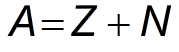Request a Tool

Atomic Mass Calculator

Atomic mass is the mass of an atom, including its protons, neutrons, and electrons

Atomic Mass (u)
0
Atomic Mass (SI)
0
Mass Number
0

Formula• A = Mass number (total number of protons and neutrons)
• Z = Number of protons in the atom
• N = Number of neutrons in the atom

Defination / Uses

The atomic mass is the mass of an atom, including its protons, neutrons, and electrons. Atomic mass is used to find the average mass of a molecules.

How to Calculate Atomic Mass ?

1. Enter the number of protons in the atom.
2. Enter the number of neutrons in the atom.
3. In Result display the atomic mass in the default unit of atomic mass units and SI unit.
4. It also display the mass number.

Use the upper given formula for manual calculations. No sign-up, registration OR captcha is required to use this tool.